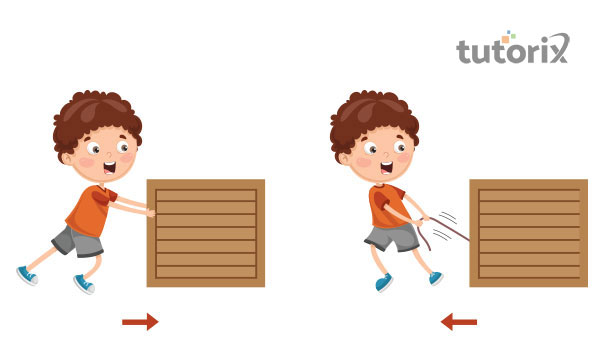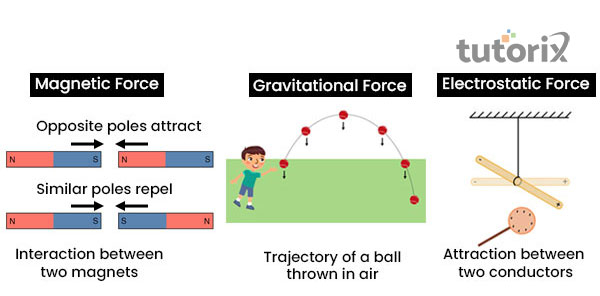# Types of Forces

## Introduction

Force mainly defines the pulling or pushing of an object and force is the most important part of the movement of objects. It can also be called the quantitative interaction between two physical bodies. The two bodies here are the environment and the object. Different types of forces can be seen in this part and among them Contact forces and Non-Contact forces are the most important. In this part Non-Contact forces is classified into three parts- Nuclear Force, Gravitational Force, and Electromagnetic Force. The Contact forces also have many variations like- Tension Force, Air Resistance Force, Frictional Force, Normal Force, Applied Force, and Spring Force. These are the main concept of the types of forces in physics.

## What is force?

Force is associated with the external effect that alters or tends to alter the state of motion of a particular body. In case the body is in motion it comes to a rest. Similarly, if a force is applied to a body that is in a state of rest the body comes to a motion. The applied force might also result in the change in the direction of the motion of the body and it also continues in alteration in the shape and size of the object (geeksforgeeks 2022). For example, the pushing and pulling of the door is done by applying a certain amount of force

Force is also classified as a vector quantity. This implies that the force has both the quantities of magnitude and direction. As per, Newton’s second law, force is defined as the product of the mass and acceleration of a body. The SI unit of force is Newton, denoted by N.

## Different types of forces

Forces are mainly classified into two types that is contact force and the non-contact forces. The first is associated with the force that involves a physical contact with the body The second does not involve any case of physical contact whatsoever.

The contact forces involve tension force, spring force and applied force.

• Tension force

This is the force that is generally applied on the ends of cable, rope or string. The force is directed to the length of the object and it pulls energy equally from both ends (oxscience 2022). Mathematically,

T = mg + ma, here T is the tension, m is mass and g is the gravitational force acting on the body

• Spring force

This is classified as the the restoring force, that is, in case a force acts on a particular body that either compress or stretches then the acting force restores the equilibrium position. Mathematically, it is mentioned as

F= kx, here F is the spring force and K is the spring constant and x is the distance of stretching and compression

• Applied force

This force is applied by one body on the other that results in an alteration in the velocity of the body or alters the direction of the body.Figure 1: Push and pull

There are three kinds of applied forces

• Push - That is the object is being pushed. It is by far the most common form of applied force in nature.
• Pull - It is associated with pulling an object. For example, pulling a door.
• Drag- This requires the active participation of two bodies. It is an event where one body drags the other.Figure 2: Non-contact forces

Non-contact force is associated with the force that does not involve any case of physical contact between one another. There are three types of non-contact forces.

• Gravitational force: This force acts between two bodies of some distinct amount of mass (sciencefacts 2022). This force is also referred an attractive force.
• Electrostatic force: The force acts between two charged bodies. For example, rubbing the scale of woollen cloth for some time and then getting it close to a piece of paper attracts the paper.
• Magnetic force: it is a non-contact force. This force acts between iron and magnets.

## Effects of force

Forces have various effects and the acting of force will accelerate or decelerate the body. It helps in giving motion to the body and it is the fact through which the speed of a moving object can increase (Zhang et al. 2018). Another important part of the effects of force is that with its application of it the speed of a moving object can also be decreased. An object’s direction can be changed through the application of force. The size and shape of an object get affected using force.

## Conclusion

Force in physics is nothing but the external cause. The cause mainly changes the state of the body. The application of force can stop the movement of the object. The application can also help in the movement of the object. The application of force also changes the direction, size and shape of an object. An example of force is pulling a window with the application of force. It is a vector quantity and it has both direction and magnitudes. The SI unit of Force is the Newton (N).

## FAQs

Q1. What is the concept of force?

Force is the movement of an object in a direction. It is the pulling or pushing of an object interacting between two physical bodies. The application of force can stop the movement of an object. It also helps in the movement of the object in a direction.

Q2. How many types of Contact forces can be seen?

In physics, Contact forces are of six types and they occur from a distance. They are Normal Force, Frictional Force, Applied Force, Air Resistance Force, Tension Force and Spring Force.

Q3. What are the types of Non-Contact forces?

There are mainly three types of Non-Contact forces present on Earth. They are Gravitational Force, Electromagnetic Force, and Nuclear Force. All the forces have their different applications.

Q4. What is meant by gravitational force?

Gravitational force refers to the force acting between objects due to presence of matter. The gravity of Earth on the objects is an example of this factor.